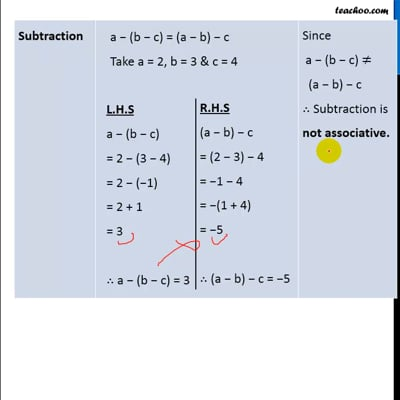Properties of Integers

Chapter 1 Class 7 Integers
Concept wise
 Operation Commutative True / False Addition a  + (b + c) = (a + b) + c True Subtraction a  − (b − c) = (a − b) − c False Multiplication a × (b × c) = (a × b) × c True Division a ÷ (b ÷ c) = (a ÷ b) ÷ c False

For Integers

Let us take three integers 2, 3, 4

 Operation Number Remark Addition a  + (b + c) = (a + b) + c Take a = 2, b = 3 & c = 4   L.H.S a + (b + c) = 2 + (3 + 4) = 2 + 7 = 9   ∴ a + (b + c) = 9   R.H.S (a + b) + c = (2 + 3) + 4 = 5 + 4 = 9   ∴ (a + b) + c = 9 Since a  + (b + c) = (a + b) + c   ∴ Addition is associative. Subtraction a − (b − c) = (a − b) − c Take a = 2, b = 3 & c = 4   L.H.S a − (b − c) = 2 − (3 − 4) = 2 − (−1) = 2 + 1 = 3   ∴ a − (b − c) = 3   R.H.S (a − b) − c = (2 − 3) − 4 = −1 − 4 = −(1 + 4) = −5   ∴ (a − b) − c = −5 Since  a − (b − c) ≠ (a − b) − c   ∴ Subtraction is not associative. Multiplication a × (b × c) = (a × b) × c Take a = 2, b = 3 & c = 4   L.H.S a × (b × c) = 2 × (3 × 4) = 2 × 12 = 24     ∴ a × (b × c) = 24   R.H.S (a × b) × c = (2 × 3) × 4 = 6 × 4 = 24   ∴ (a × b) × c = 24 Since  a × (b × c) = (a × b) × c   ∴ Multiplication is associative. Division a ÷ (b ÷ c) = (a ÷ b) ÷ c Take a = 2, b = 3 & c = 4   L.H.S a ÷ (b ÷ c) = 2÷(3÷4) = 2÷(3/4) = 2×4/3 = 8/3   R.H.S (a ÷ b) ÷ c = (2÷3) ÷4 = (2/3)÷4 = 2/3×1/4 = 1/6   a ÷ (b ÷ c) ≠ (a ÷ b) ÷ c Since a ÷ (b ÷ c) ≠ (a ÷ b) ÷ c   ∴ Division is not associative.This video is only available for Teachoo black users